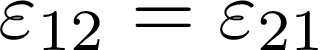# SymmetricSecondRankTensor indexing (Straintensor)

Hi,

I tried calculating Straintensor with ITKStrain Module http://insight-journal.org/browse/publication/984 from a 2D DisplacementField (256,256,2). The output is a SymmetricSecondRankTensor. I converted to numpy array and get an (256,256,3)- shaped array.

My question concerns the indexing. Due to symmetry reasons a 2D-SymmetricSecondRankTensor would look like:

a b
b c

hence only one “b” is stored (leading to dimension 3 in numpy array). But the question remains, which index (especially in numpy) represents which value. I read the Documentation of ITK::SymmetricSecondRankTensor, but unfortunatly i did not understand itCan anyone help me?

Thanks in advance.

1 Like

For a 256x256 displacement image you get a 256x256 tensor image. From my reading of the documentation, the three indices of the third dimension should be [a,b,c] from

``````a b
b c
``````

matrix of the tensor.

1 Like

Thanks alot. I am going to stick to it :-).

Yes, the strain tensor isbut, since strain is a symmetric tensor,